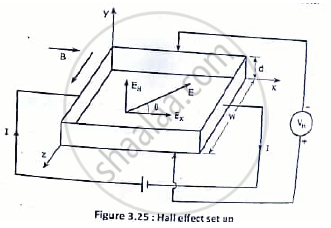# State the Hall Effect. Derive the Expression for Hall Voltage and Hall Coefficient with Neat Diagram. - Applied Physics 1

State the Hall effect. Derive the expression for Hall voltage and Hall coefficient with neat diagram.

State the Hall effect. Derive the expression for Hall coefficient with neat diagram.

#### Solution

if a current carrying conductor or semiconductor is placed in a transverse magnetic field, a potential difference is developed across the specimen in a direction perpendicular to both the current and magnetic field. The phenomenon is called HALL EFFECT.
As shown consider a rectangular plate of a p-type semiconductor of width ‘w’ and thickness ‘d’ placed along x-axis. When a potential difference is applied along its length ‘a’ current ‘I’ starts flowing through it in x direction.
As the holes are the majority carriers in this case the current is given by

I = nh Aevd  ……………………………(1)

where n= density of holes

A  = w×d =  crosss ectional area of the specimenVd = drift velocity of the holes.

The current density is

J = I/ A = n_h  ev_d …………………..(2)

The magnetic field is applied transversely to the crystal surface in z direction. Hence the holes experience a magnetic force

Fm = evdB …………………………….(3)
In a downward direction. As a result of this the holes are accumulated on the bottom surface of the specimen.
Due to this a corresponding equivalent negative charge is left on the top surface.
The separation of charge set up a transverse electric field across the specimen given by,

E_ = (V_H)/ d …………………………..(4)

Where VH is called the HALL VOLTAGE and EH the HALL FIELD.

In equilibrium condition the force due to the magnetic field B and the force due to the electric field
EH acting on the charges are balanced. So the equation (3)

eE_H = ev_d B
E_H = v_d B ……………………………….(5)

Using equation (4) in the equation (5)
V_H = v_d B d ………………………….(6)

From equation (1) and (2), the drift velocity of holes is found as

v_d =I/ (en_h A) = J/(en_h) ……………………..(7)

Hence hall voltage can be written as V_H =(IBd)/( en. A) = ( J_xBd)/( en.) as

An important parameter is the hall coefficient defined as the hall field per unit current density per unit magnetic induction.

R_H = (E_H)/(J_xB)

Concept: Hall Effect
Is there an error in this question or solution?